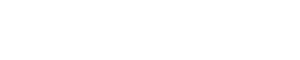Department of MathematicsFirst-Year Courses in the Graduate Program

The courses described below are the core of the first year graduate program. The program undergoes regular reevaluation and change, so the list of topics is only approximate, and the content of the courses also varies from year to year at the discretion of the instructor.

Analysis

Math 312: Analysis I

Measure theory, integration and $$L^p$$ spaces, differentiation, basic functional analysis. Additional topics may be discussed depending on the instructor.

Taught in Fall 2016 by Luis Silvestre.

Prerequisite: Graduate student status or instructor consent

Math 313: Functional Analysis

Weak convergence, compact operators, spectral theory, Sobolev spaces, and some applications. Additional topics may be discussed depending on the instructor.

Taught in Winter 2017 by Charles Smart.

Prerequisite: Math 312

Math 314: Complex Analysis and Topics in Analysis

Basic complex analysis, Cauchy theorem in the homological formulation, residues, meromorphic functions, Mittag-Leffler theorem, Gamma and Zeta functions, analytic continuation, mondromy theorem, the concept of a Riemann surface, meromorphic differentials, divisors, Riemann-Roch theorem, compact Riemann surfaces, uniformization theorem, Green functions, hyperbolic surfaces, covering spaces, quotients.

Taught in Spring 2017 by Gregory Lawler.

Prerequisite: Math 313

Geometry and Topology

Math 317: Algebraic Topology

Fundamental group, covering space theory and Van Kampen's theorem (with a discussion of free and amalgamated products of groups), homology theory (singular, simplicial, cellular), cohomology theory, Mayer-Vietoris, cup products, Poincare Duality, Lefschetz fixed-point theorem, some homological algebra (including the Kunneth and universal coefficient theorems), higher homotopy groups, Whitehead's theorem, exact sequence of a fibration, obstruction theory, Hurewicz isomorphism theorem.

Taught in Fall 2016 by Danny Calegari.

Prerequisite: Undergraduate analysis, algebra, and (preferably) topology.

Math 318: Differential Topology

Definition of smooth manifolds, tangent and cotangent bundles, vector bundles. Inverse and implicit function theorems. Sard's theorem and the Whitney embedding theorem. Degree of maps. Vector fields and flows, transversality, and intersection theory. Frobenius' theorem, differential forms and the associated formalism of pullback, wedge product, integration, etc. Cohomology via differential forms, and the de Rham theorem. Further topics may include: compact Lie groups and their representations, Morse theory, cobordism, and differentiable structures on the sphere.

Taught in Winter 2017 by Amie Wilkinson.

Prerequisite: Math 317

Math 319: Differential Geometry

Riemannian metrics, connections and curvature on vector bundles, the Levi-Civita connection, and the multiple interpretations of curvature. Geodesics and the associated variational formalism (formulas for the 1st and 2nd variation of length), the exponential map, completeness, and the influence of curvature on the topological structure of a manifold (positive versus negative curvature). Lie groups. The Chern-Weil description of characteristic classes, the Gauss-Bonnet theorem and possibly the Hodge Theorem.

Taught in Spring 2017 by Andre Neves.

Prerequisite: Math 318

Algebra

Math 325: Representation Theory

Representation theory of finite groups, including symmetric groups and finite groups of Lie type; group rings; Schur functors; induced representations and Frobenius reciprocity; representation theory of Lie groups and Lie algebras, highest weight theory, Schur–Weyl duality; applications of representation theory in various parts of mathematics.

Taught in Fall 2016 by Victor Ginzburg.

Prerequisite: Graduate student status or instructor consent

Math 326: Commutative Algebra and Algebraic Geometry

This course will explain the dictionary between commutative algebra and algebraic geometry. Topics will include the following. Commutative ring theory: Noetherian property; Hilbert Basis Theorem; localization and local rings; etc. Algebraic geometry: affine and projective varieties, ring of regular functions, local rings at points, function fields, dimension theory, curves, higher-dimensional varieties.

Taught in Winter 2017 by Matthew Emerton.

Prerequisite: Math 325

Math 327: Topics in Algebra

According to the inclinations of the instructor, this course may cover: algebraic number theory; homological algebra; further topics in algebraic geometry and/or representation theory.

Taught in Spring 2017 by Kazuya Kato.

Prerequisite: Math 326